Next: Photons Up: QED Processes Previous: Theorem 6

# Coulomb Scattering of Positrons

We now consider the scattering of positrons in a Coulomb field. We expect the form to be similar to the Coulomb scattering of an electron.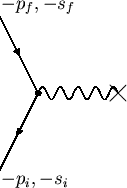The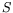-matrix for the process in figure 7.3 is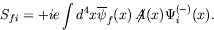(7.80)

Here the incoming state is in the future and is to be interpreted as a negative-energy electron of four-momentum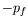running backward in time. Putting in plane waves to lowest order, the wave function is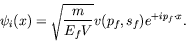(7.81)

Similarly, the outgoing state is the negative-energy electron running backward into the past. Its wave function is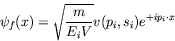(7.82)

representing the incident positron with momentum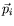and polarization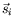before the scattering. The-matrix becomes (cf equation 7.30)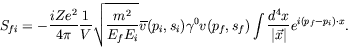(7.83)

By the same calculation as in equation 7.45, we find the differential cross-section is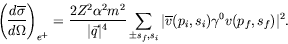(7.84)

Again the spin sum may be reduced to a trace, using the relation for positron spinors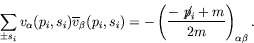(7.85)

The first minus sign comes from the normalization of the negative-energy spinors and the relative minus sign of the two terms comes from the negative-energy projection operator.

The differential cross-section now becomes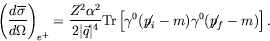(7.86)

This is the same as the result of equation 7.50 for the electron with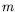replaced by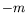. Since our answer for electron scattering was even in, this shows that the positron scattering cross-section is equal to the electron scattering cross-section in lowest order of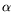.

We could have anticipated this result from charge conjugation invariance. We could equally well write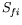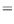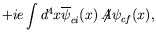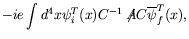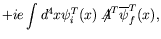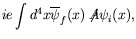(7.87)

which leads to the same results as before. In this picture the positron runs forward in time and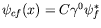is the wave function of the initial positron.

Previously we saw that for each solution of the electron in the potential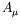there is a corresponding solution of the positron in the potential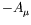, that is, the scattering of an electron from the potential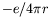is the same as that of a positron from potential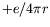; however, since the calculated cross-section depends only on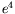, the sign ofdoes not matter. This is not true for the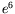correction which comes from the product of the first- and second-order scattering amplitudes, which have opposite signs for electrons and positrons.

We may also observe that the positron cross-section is obtained from that of the electron by replacing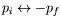; this is a general feature of positron theory called the substitution rule'', which is closely related to the propagator picture we have developed.Next: Photons Up: QED Processes Previous: Theorem 6
Douglas M. Gingrich (gingrich@ ualberta.ca)
2004-03-18